• 等高线图（contour map） 是可视化二维空间标量场的基本方法，可以将三维数据使用二维的方法可视化，同时用颜色视觉特征表示第三维数据，如地图上的等高线、天气预报中的等压线和等温线等。假设 f(x,y)f(x, y)f(x,y)...
简介
等高线图（contour map） 是可视化二维空间标量场的基本方法，可以将三维数据使用二维的方法可视化，同时用颜色视觉特征表示第三维数据，如地图上的等高线、天气预报中的等压线和等温线等。假设 $f(x, y)$ 是在点 $(x, y)$ 处的数值，等值线是在二维数据场中满足 $f(x, y)=c$ 的空间点集按一定的顺序连接而成的线。数值为c的等值线可以将二维空间标量场分为两部分：如果 函数大于c，则该点在等值线内；反之，则该点在等值线外。
#所需的包
library(reshape2) #数据处理相关
library(ggplot2) # 绘图相关
library(grDevices) #绘图颜色相关
library(RColorBrewer)#绘图颜色相关
library(directlabels) #等高线相关

数据介绍
数据为z，breaks_lines为之后需要绘图的间隔准备。
z <- as.matrix(read.table("等高线.txt",header=TRUE))
colnames(z) <- seq(1,ncol(z),by=1) #列名设置
max_z <- max(z)
min_z <- min(z)
breaks_lines <- seq(min(z),max(z),by=(max_z-min_z)/10)
map <- melt(z) #这个函数来自reshape2（处理数据的包）介绍一下这个函数，以及相关函数
dim(map)
colnames(map)<-c("Var1","Var2","value")

reshape2包中的melt()函数可将数据从左图转换呈右图的数据形式（下面图片可滑动查看！）。

含义：转换前第一行在转换后第一列为1，对应位置在第二列，第三列是对应值。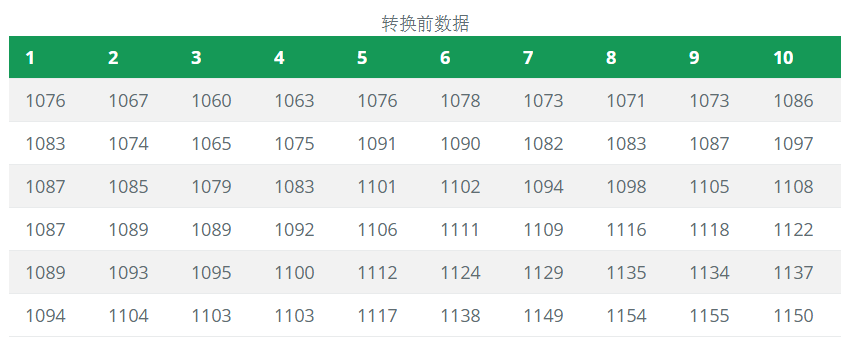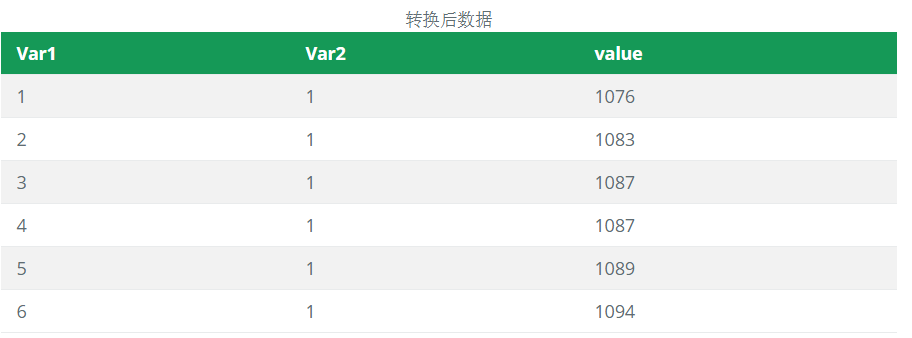设置颜色调色盘，以后详细讲解。
rf <- colorRampPalette(rev(brewer.pal(11,'Spectral')))
colormap <- colorRampPalette(rev(brewer.pal(11,'Spectral')))(32)

热力分布图
使用ggplot2包中的geom_tile()或者geom_raster()绘制热
力分布图。其主要区别在于geom_raster()函数中存在interpolate=TRUE/FALSE这个参数，决定是否对热力图进行平滑处理。
这里使用geom_tile()进行演示，将三维数据(x,y,z)中(x,y)表示位置信息，z映射到颜色。这里的scale_fill_gradientn()将颜色填充呈n个梯度。

拓展：scale_*_gradient创建一个双色梯度(低-高)，scale_*_gradient2创建一个渐变的颜色梯度(低-中-高)，scale_*_gradientn创建一个n色梯度。

ggplot(map,aes(x=Var1,y=Var2,z=value))+
geom_tile(aes(fill=value))+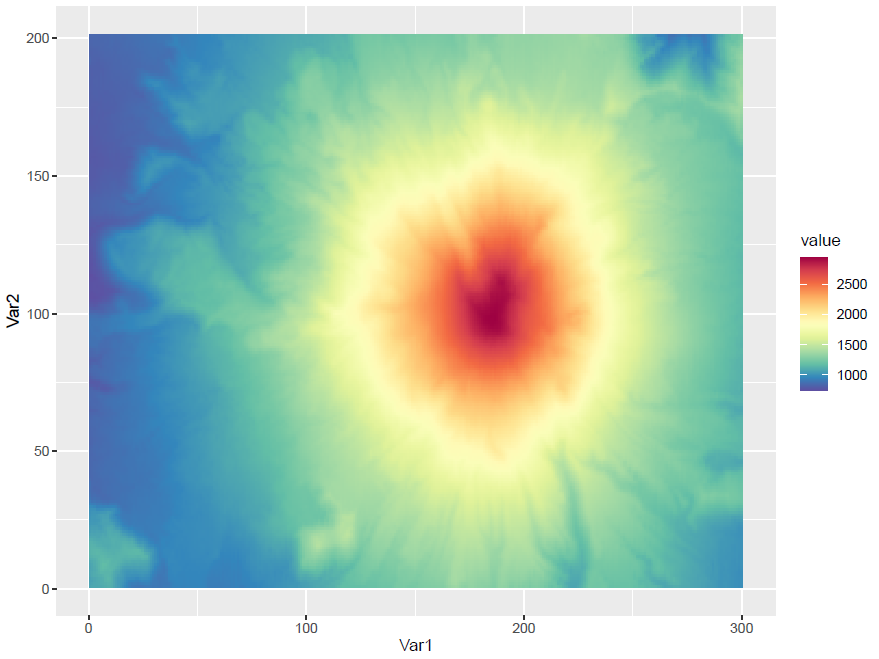添加等高线
使用geom_contour()在上图基础上添加等高线，同一轮廓上的数值相同。
ggplot(map,aes(x=Var1,y=Var2,z=value))+
geom_tile(aes(fill=value))+#根据高度填充
geom_contour(breaks=breaks_lines,color="black")+#
labs(x="X-Axis",y="Y-Axis",fill="Z-Value")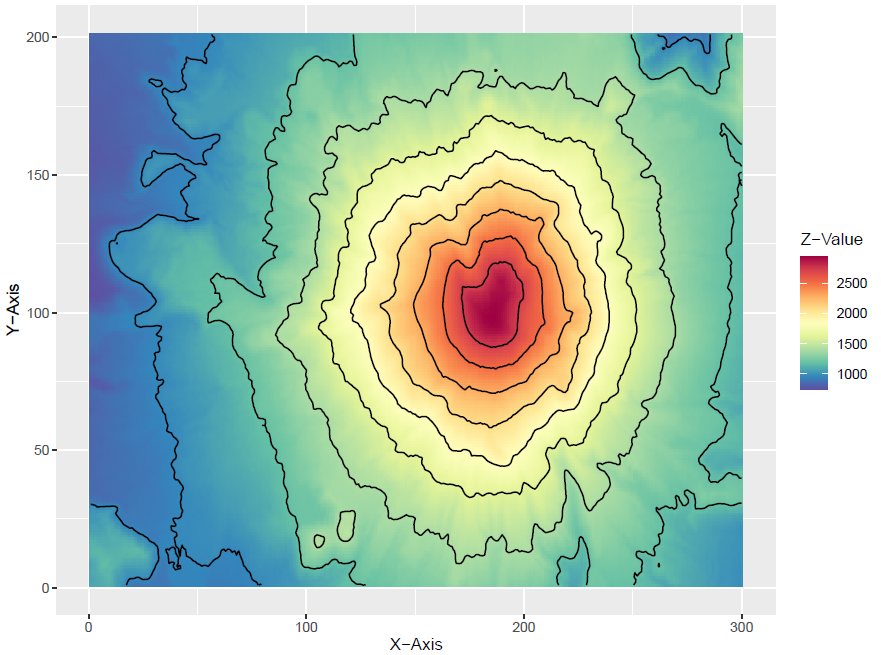设置主题
对主题进行稍微的调整。改变x轴题目(axis.title,大小为15，字体形式为常规体face="plain"，颜色黑色)，x轴文字(axis.text),图例标题(legend.title)图例文字,(legend.text),(图例背景legend.background),图例位置(legend.position)
Contour <- ggplot(map,aes(x=Var1,y=Var2,z=value))+
geom_tile(aes(fill=value))+#根据高度填充
geom_contour(breaks=breaks_lines,color="black")+#
labs(x="X-Axis",y="Y-Axis",fill="Z-Value")+
theme(
axis.title = element_text(size=15,face="plain",color="black"),
axis.text = element_text(size=13,face="plain",color="black"),
legend.title = element_text(size=13,face="plain",color="black"),
legend.text = element_text(size=11,face="plain",color="black"),
legend.background = element_blank(),
legend.position = c(0.15,0.2)
)
Contour


拓展：face还可以设置其他字体形式：plain（常规体）、bold（粗体）、italic（斜体）、bold.italic（粗斜体）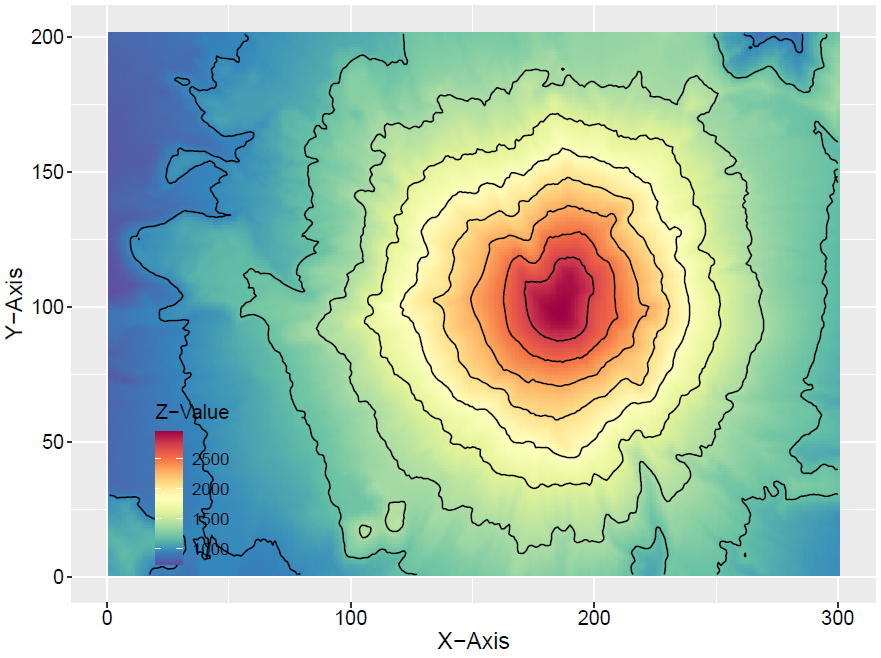添加等高线的具体数值
在上面的图基础上，利用directlabels包的direct.label()添加等高线的具体数值，从而不需要颜色映射的图例，同一轮廓上的数值相同。

作用：在二维屏幕上，等高线可以有效地表达相同数值的区域，揭示走势和陡峭程度及两者之间的关系，寻找坡、峰、谷等形状。

direct.label(Contour, list("bottom.pieces", cex=0.8,
fontface="plain", fontfamily="serif", colour='black'))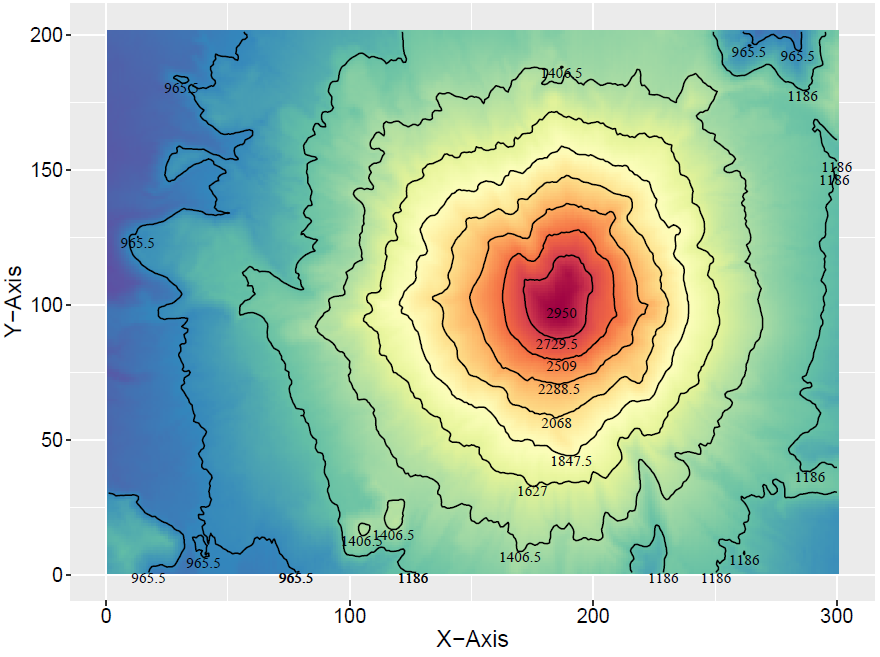以上对绘制等高线进行了详细介绍了，如果还需进一步了解，可参考一下网上免费开源资料：
Using 2D Contour Plots within {ggplot2} to Visualize Relationships between Three Variables
A guide to contour maps
Contour Plots in R。
本篇视为《R语言数据可视化之美》学习笔记，并进行函数详细介绍与解释，其他可视化图可参考在菜单命令中搜索得到。对应代码与相关数据，请在我的github中获取（文末原文）。
喜欢请一键三连，创作不易，感恩不尽。


展开全文可视化 数据可视化 机器学习
• Excel等高线图.rar,等高线图可用来制作地理、温度等表示高低变量和位置关系的图表，见本例。Excel应用
• 等高线图 对轮廓图的支持。 如何安装？ 要安装contour-plot ，请转到图像中的Playground（Ctrl + OW）并执行以下Metacello脚本（选择它并按Do-it按钮或Ctrl + D）： Metacello new baseline: ' AIContourPlot ' ;...Smalltalk
• 主要介绍了用matplotlib画等高线图详解，具有一定借鉴价值，需要的朋友可以参考下。
• 介绍三种绘制云图或等高线图的算法。点距离反比插值、双线性插值和面距离反比+双线性插值。
• ## 等高线图：

千次阅读 2018-06-29 22:33:11
最近想寻找一种能利用已有数据画出地理位置分布图的方法，在看Vamei写的博客 绘图: matplotlib核心剖析 学习matplotlib绘图的过程中找到了名为“contour”(等高线图)的绘图方式等高线图：等高线地图就是将地表高度...
最近想寻找一种能利用已有数据画出地理位置分布图的方法，在看Vamei写的博客 绘图: matplotlib核心剖析 学习matplotlib绘图的过程中找到了名为“contour”(等高线图)的绘图方式等高线图：等高线地图就是将地表高度相同的点连成一环线直接投影到平面形成水平曲线。不同高度的环线不会相合，除非地表显示悬崖或峭壁才能使某处线条太密集出现重叠现像，若地表出线平坦开阔的山坡，曲线间之距离就相当宽。 (ps：从百度百科上扒来的一段话。话说百科上面错别字还是不少的，最后一行的“出现”错写成了“出线”)对我这种学计算机的工科生来说，接触等高线图大部分（甚至可以说唯一）的机会就在初中和高中时的地理课堂上了，such as ：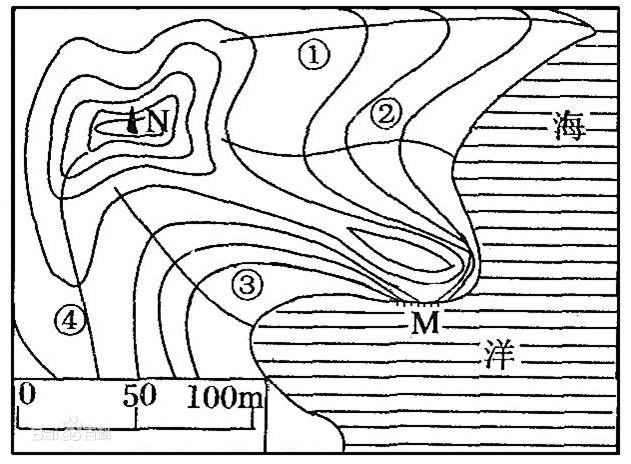下面来介绍怎么用matplotlib画简单的等高线图：代码的参考来自于 绘图: matplotlib核心剖析 和 matplotlib的基本用法(九)——绘制等高线图# -*- coding:utf-8 -*-
#引入numpy库和matplotlib库
import numpy as np
import matplotlib.pyplot as plt

# 定义等高线图的横纵坐标x，y
#从左边取值为从 -3 到 3 ，各取5个点，一共取 5*5 = 25 个点
x = np.linspace(-3, 3, 5)
y = np.linspace(-3, 3, 5)
# 将原始数据变成网格数据
X, Y = np.meshgrid(x, y)

# 各地点对应的高度数据
#Height是个 5*5 的数组，记录地图上 25 个点的高度汇总
Height = [[0,0,1,2,2],[0,-2,-2,1,5],[4,2,6,8,1],[3,-3,-3,0,5],[1,-5,-2,0,3]]

# 填充颜色
plt.contourf(X, Y, Height, 10, alpha = 0.6, cmap = plt.cm.hot)
# 绘制等高线
C = plt.contour(X, Y, Height, 10, colors = 'black', linewidth = 0.5)
# 显示各等高线的数据标签
plt.clabel(C, inline = True, fontsize = 10)
plt.show()1234567891011121314151617181920212223画出的图如下：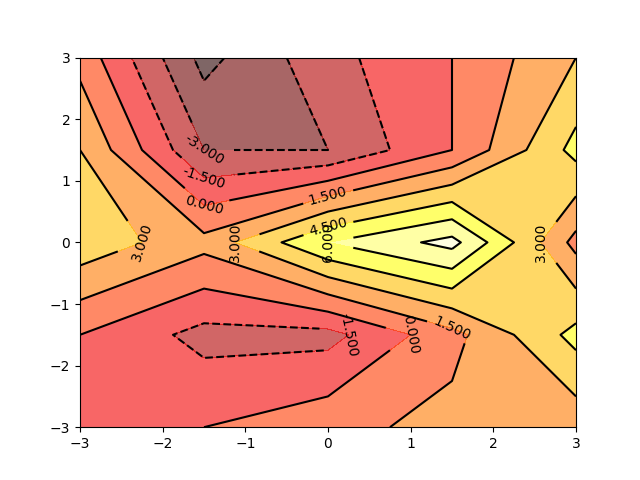由于这25个取样点的高度数值都是我凭感觉设置的，所以制作出来的效果不是很好，但拿来说明问题基本足够了。下面简单解释一下代码中主要的函数 contourf 和 contour 中参数的设置：1.contourf 填充颜色函数前三个参数 X, Y, Height 用来引进点的位置和对应的高度数据；数字 10 代表将等高线图分成10块（这里不是硬性要求，但数值过小会造成部分分区颜色区分度不高）；alpha = 0.6 用来设置填充颜色的范围，alpha取值为 [0,1) 。alpha=0时，画出的是无色图，alpha越接近1，颜色的搭配就越向深色风格过渡，如下面几张图所示：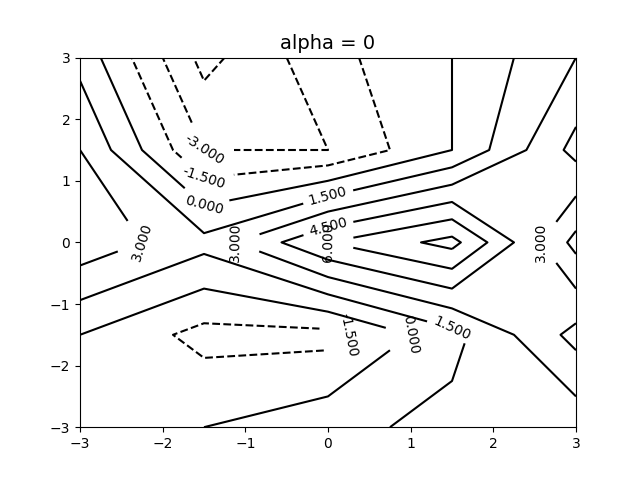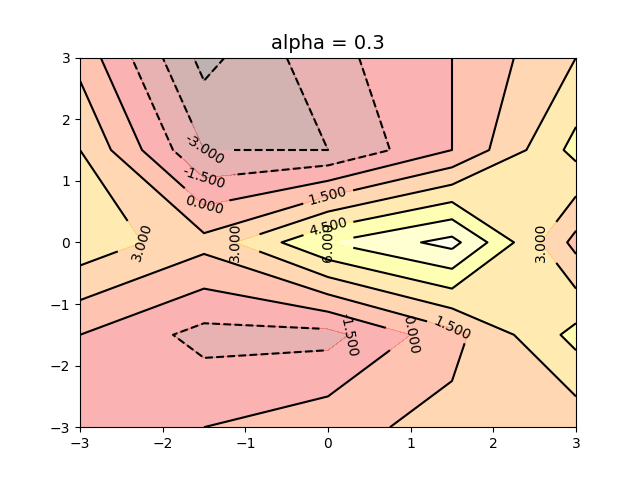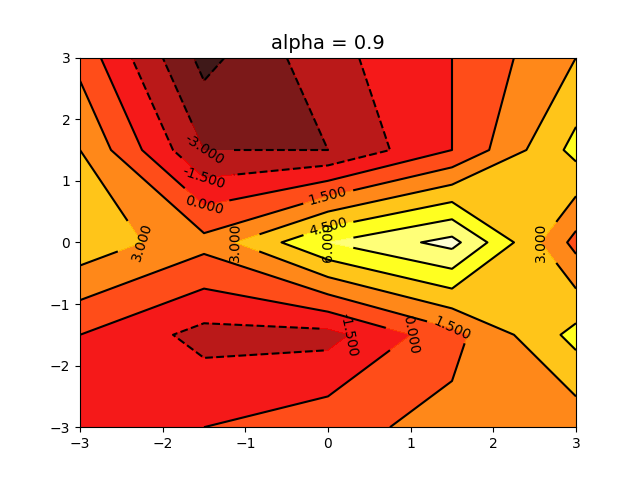2.contour 绘制等高线函数前面的参数跟contourf一样；colors = ‘black’ 代表线条颜色是黑色；linewidth = 0.5 代表线条粗细.如果遇到不能靠单纯输入各点高度值的情况，就需要将Height数组变成一个以横纵坐标X和Y为参数的高度计算函数：def f(x, y):
return x * y #这里的函数是我简单定义的，要根据现实情况设置符合实际的函数12总代码：# -*- coding:utf-8 -*-
#引入numpy库和matplotlib库
import numpy as np
import matplotlib.pyplot as plt

# 定义等高线图的横纵坐标x，y
#从左边取值为从 -3 到 3 ，各取5个点，一共取 5*5 = 25 个点
x = np.linspace(-3, 3, 5)
y = np.linspace(-3, 3, 5)
# 将原始数据变成网格数据
X, Y = np.meshgrid(x, y)

# 定义等高线高度函数
def f(x, y):
return x * y

# 填充颜色
plt.contourf(X, Y, f(X,Y), 10, alpha = 0.5, cmap = plt.cm.hot)
# 绘制等高线
C = plt.contour(X, Y, f(X,Y), 10, colors = 'black', linewidth = 0.5)
# 显示各等高线的数据标签
plt.clabel(C, inline = True, fontsize = 10)
plt.show()1234567891011121314151617181920212223画出的图如下：（莫名地有一种对称的美感 2333）可以在最后的 plt.show() 前加如下两行，将横纵坐标隐藏：# 去除坐标轴
plt.xticks(())
plt.yticks(())123效果如下：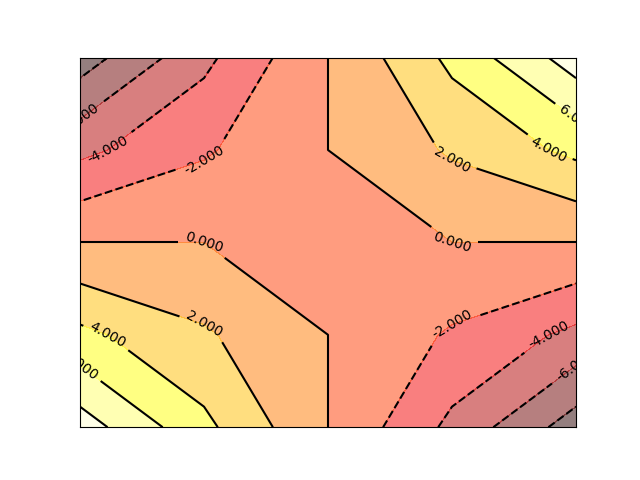以上就是用matplotlib画等高线图的基本步骤，以后有机会的话得尝试一下如何绘制功能更强大、更符合实际应用的等高线图。 — 2:55 pm, 2017/6/25.画等高线的代码如下：?1234567891011121314151617181920import numpy as npimport pandas as pdimport matplotlib.pyplot as plt # 计算x,y坐标对应的高度值def f(x, y): return (1-x/2+x**5+y**3) * np.exp(-x**2-y**2) # 生成x,y的数据n = 256x = np.linspace(-3, 3, n)y = np.linspace(-3, 3, n) # 把x,y数据生成mesh网格状的数据，因为等高线的显示是在网格的基础上添加上高度值X, Y = np.meshgrid(x, y) # 填充等高线plt.contourf(X, Y, f(X, Y))# 显示图表plt.show()上述代码显示的图形为：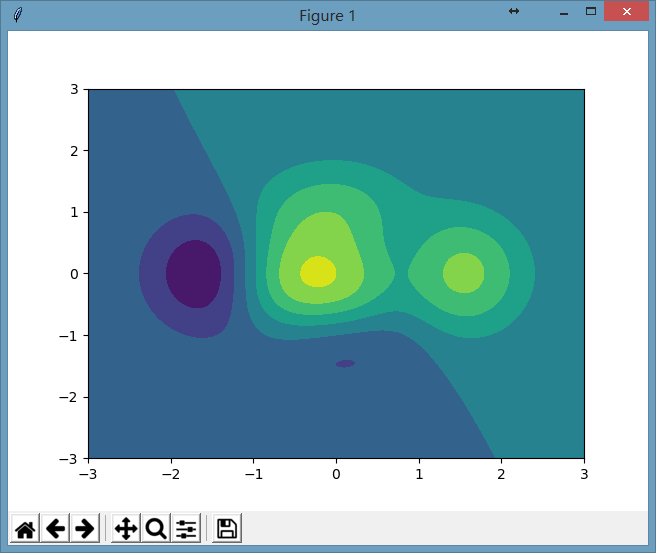这颜色有点太冷了，我们想显示热力图，那只要在plt.contourf()函数中添加属性cmap=plt.cm.hot就能显示热力图，其中cmap代表为color map，我们把color map映射成hot(热力图)，此处关键代码为：?12# 填充等高线plt.contourf(X, Y, f(X, Y), cmap=plt.cm.hot)显示的图为：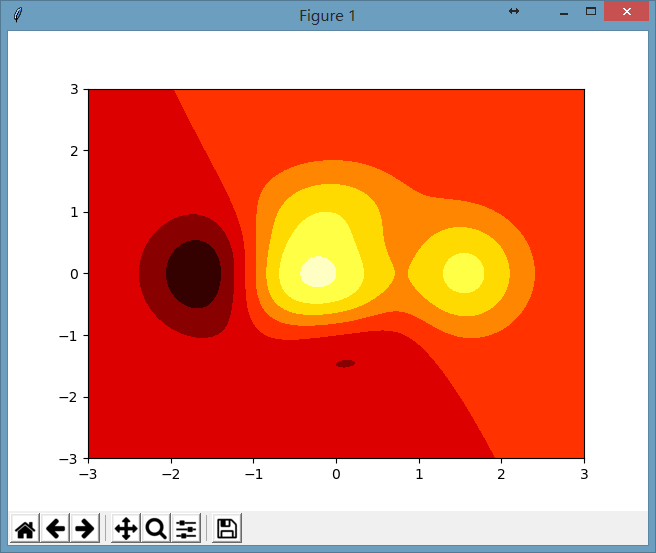是否显示得挺热的。：）上面是用plt.contourf()填充了等高线，但还有一种方式是可以直接显示等高线，而不是填充的方式，例如：?1C = plt.contour(X, Y, f(X, Y), 20)这里20代表的是显示等高线的密集程度，数值越大，画的等高线数就越多。这样显示的图形为：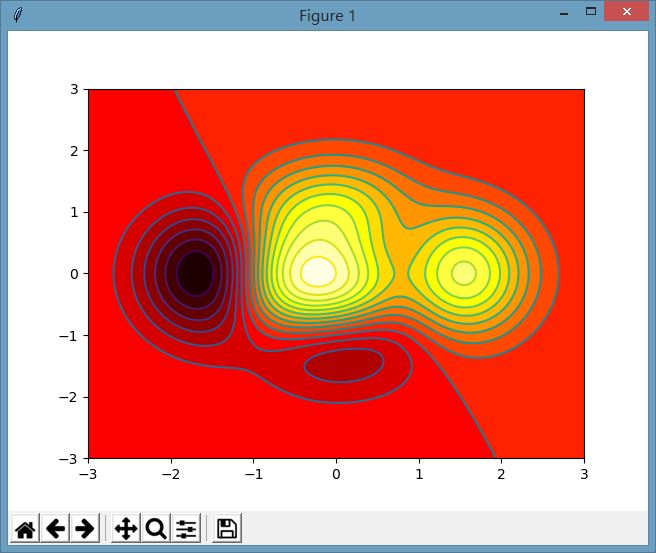展开全文• 数据环形图+等高线图，环形数据 程序演示视频，进入主页观看
• 这是如何在 MATLAB:registered: 中创建等高线图的示例。 阅读 MATLAB 文档中的“轮廓”函数。 有关更多示例，请转到 MATLAB 绘图库 - http://www.mathworks.com/discovery/gallery.htmlmatlab
• 如果你想知道Z的变化是如何作为两个输入X和Y的函数，如Z=f(X,Y)，等高线图是合适的。两个变量函数的等高线或等值线是一条曲线，该函数的数值是恒定的。 独立变量x和y通常被限制在一个叫做meshgrid的规则网格中。...
目录
等高线图（有时也叫水平图）是在二维平面上显示三维曲面的一种方法。它将y轴上的两个预测变量X Y和一个响应变量Z绘制成等值线图。这些等高线有时被称为z切片或等值线响应值。
如果你想知道Z的变化是如何作为两个输入X和Y的函数，如Z=f(X,Y)，等高线图是合适的。两个变量函数的等高线或等值线是一条曲线，该函数的数值是恒定的。
独立变量x和y通常被限制在一个叫做meshgrid的规则网格中。numpy.meshgrid可以用x值的数组和y值的数组创建一个矩形网格。
Matplotlib API包含contour()和contourf()函数，分别绘制等高线和填充等高线。这两个函数都需要三个参数x,y和z。
import numpy as np
import matplotlib.pyplot as plt
xlist = np.linspace(-3.0, 3.0, 100)
ylist = np.linspace(-3.0, 3.0, 100)
X, Y = np.meshgrid(xlist, ylist)
Z = np.sqrt(X**2 + Y**2)
fig,ax=plt.subplots(1,1)
cp = ax.contourf(X, Y, Z)
fig.colorbar(cp) # Add a colorbar to a plot
ax.set_title('Filled Contours Plot')
#ax.set_xlabel('x (cm)')
ax.set_ylabel('y (cm)')
plt.show()

显示结果如下：X, Y = np.meshgrid(x, y) 代表的是将x中每一个数据和y中每一个数据组合生成很多点,然后将这些点的x坐标放入到X中,y坐标放入Y中,并且相应位置是对应的，如下所示：
如: x = [1, 2, 3, 4]
y = [7, 8, 9]

x和y中的每一个元素组合生成
[[[1, 7], [2, 7], [3, 7], [4, 7]],
[[1, 8], [2, 8], [3, 8], [4, 8]],
[[1, 9], [2, 9], [3, 9], [4, 9]]]

然后
再分别放入X和Y中
X = [[1, 2, 3, 4],
[1, 2, 3, 4],
[1, 2, 3, 4]]

Y = [[7, 7, 7, 7],
[8, 8, 8, 8],
[9, 9, 9, 9],]


coutour([X, Y,] Z,[levels], **kwargs)，是来绘制等高线的，contour和contourf都是画三维等高线图的，不同点在于contour() 是绘制轮廓线，contourf()会填充轮廓。除非另有说明，否则两个版本的函数是相同的。具体实例见这里。


展开全文• MATLAB --等高线图
等高线图
假如我们要绘制某个函数：x的取值范围在-5到5之间，y的取值范围在-3到3之间，增量为0.1：
>> [x,y] = meshgrid(-5:0.1:5,-3:0.1:3);

其中meshgrid命令是用来产生网格的
我们也可以先指定增量，然后调用meshgrid命令来生成网格，例如：
>> x = [-5:0.1:5];
>> y = [-3:0.1:3];
>> [X,Y] = meshgrid(x,y);

只不过我们把小写的x,y当成了中间变量，最后的值是X，Y。
假如我们绘制的函数是：那么我们输入以下命令：
>> z = X.^2 + Y.^2;

现在我们调用contour命令：
>> contour(X,Y,z)

产生的图像：我们还可以为这个图像添加更多的内容：

标签
等高线之间的宽度
线宽
线型
线的颜色
需不需填充
以上属性的修改可以使用set命令修改
需要注意的是，我们返回当前图像的属性时，因为是二维的网格，所以就有两个参数，我们可以用矩阵的形式表示：

>> [C,h] = contour(X,Y,z)

其中共有两个参数：

C
其中第一个参数表示x,y坐标的矩阵，是一个二维数组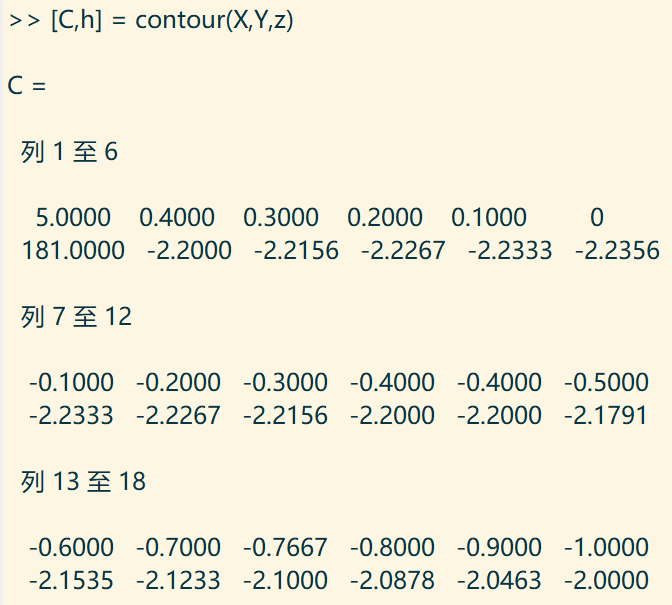h
h表示一些属性：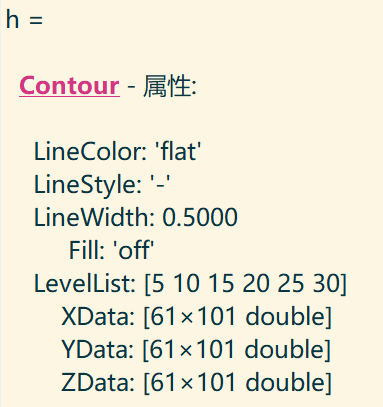当然，这两个参数的名字我们也可用其它字母替代，只要放在一个数组里面就行。
我们调用set命令：

>> set(h,'showtext','on','textstep',get(h,'levelstep')*2)

这一次，我们为等高线图添加了标签：让我们绘制另一个函数：>> [x,y] = meshgrid(-5:0.1:5,-3:0.1:3);
>> z = cos(x).*sin(y);
>> [C,h] = contour(x,y,z);
>> set(h,'showtext','on','textstep',get(h,'levelstep')*2)我们还可以调用contour3命令来把等高线画成三维的。如果我们调用contour3(z,n)，那么他将产生有n个级别的等高线：
>> contour3(z,10)这一次我们绘制另一个函数，x和y的取值范围相同：>> [x,y] = meshgrid(-2:0.1:2)
>> z  = y.*exp(-x.^2-y.^2);
>> contour(x,y,z),xlabel('x'),ylabel('y')


当两个独立变量在同一范围的时候，可以把：
[x,y] = meshgrid(x)下面我们绘制三维：
仅仅输入：

>> contour3(x,y,z)

便得到：展开全文matlab
• 因此，如何生成成形零件的等高线图是该工艺的关键技术之一。本文对板料渐进成形工艺等高线图的生成方法进行了研究，提出一种基于 Matlab拟合Bezier曲面并快速生成等高线图的方法。对理论抛物面和飞机翼面的仿真研究...
• 地质类专业基础课 构造地质学 实习七 构造等高线图编制及分析 一实习目的 (1)学会根据岩层标高(或埋深)资料编绘构造等高线图 (2)学会认识和分析构造等高线所反映的构造形态 二方法说明 构造等高线图是用等高线来反映...
• ## matplotlib等高线图-

千次阅读 2017-12-14 11:10:37
等高线图是在地理课中讲述山峰山谷时绘制的图形，在机器学习中也会被用在绘制梯度下降算法的图形中。 因为等高线的图有三个信息：x, y以及x,y所对应的高度值。 这个高度值的计算我们用一个函数来表述： # ...
• 利用d3.js实现画等值线图、等值云图、等值云图的demo，浏览器直接运行即可，其中通过反距离加权法（IDW）先进性插值，后面再通过conrec.js这个工具类将等值线图所需的数据生成出来，之后再通过d3.js将等值线图、等值...
• MATLAB使用surf、contour3灵活绘制投影图、等高线图，显示在指定高度平面
• 在机器学习的随机梯度下降中涉及了等高线图用以形象的描述每次迭代的步距、方向等，这也正是它的用武之地。仅仅学习其中的皮毛。 君子性非异也，善假于物也 import numpy as np import matplotlib.pyplot as plt #...matplotlib python contours
• 最近需要用到Matlab来绘制三维的地形图等，即通过已知的山地海拔数据，利用Matlab软件使用插值法绘制三维曲面图、等高线图、伪彩色图、散点图。 一、Matlab插值 插值就是在已知数据之间计算估计值的过程,是一种实用...matlab 矩阵
• 1.普通等高线 >>[x,y]=meshgrid(-5:0.1:5,-3:0.1:3); >>z=x.^2+y.^2; >>contour(x,y,z) 给普通等高线加标签 >>[C,h]=contour(x,y,z); >>set(h,'ShowText','on','TextStep',get...
• matlab程序里生成等高线图的高程点怎么在图上标注高程啊？matlab
• ## Contours 等高线图

千次阅读 2018-07-29 22:10:00
Contours 等高线图 1、画等高线 数据集即三维点 (x,y) 和对应的高度值，共有256个点。高度值使用一个 height function f(x,y) 生成。 x, y 分别是在区间 [-3,3] 中均匀分布的256个值，并用meshgrid在二维平面...
• matplotlib 中的二维等高线图和频次直方图 import matplotlib.pyplot as plt import numpy as np plt.style.use('seaborn-white') %matplotlib inline def f(x,y): return np.sin(x)**10+np.cos(10+x*y)*np.cos(x) ...python numpy
• 已经将电机的工作点在等高线图上已撒点了，如何将某个区域的点的个数统计出来？比如一共有多少个点落在80-85%效率区间？c++ 开发语言 c语言...